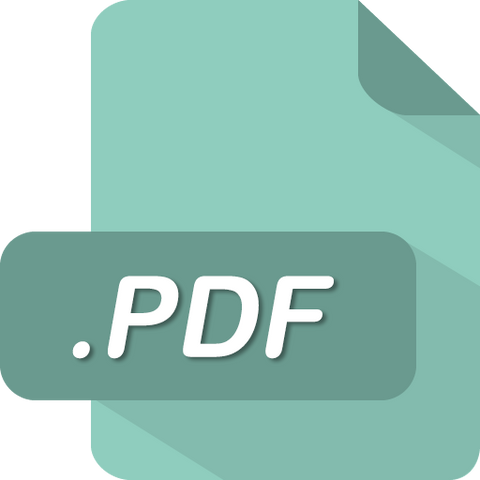# BUSI 530 BUSI530 Module 5 Homework 5 (Liberty University)

soffix

• \$14.99

BUSI530 Module 5 Homework 5 (Liberty University)

1. Problem 11­1 Rate of Return (LO2)

A stock is selling today for \$50 per share. At the end of the year, it pays a dividend of \$2 per share and sells for \$56.

2. Problem 11­3 Real versus Nominal Returns (LO2)

You purchase 100 shares of stock for \$40 a share. The stock pays a \$2 per share dividend at year­end. What is the rate of return on your investment for the end­of­year stock prices listed below? What is your real (inflation­adjusted) rate of return? Assume an inflation rate of 3%. (Leave no cells blank ­ be certain to enter "0" wherever required. Negative values should be indicated by a minus sign. Do not round intermediate calculations. Round your "Real Rate of Return" answers to 2 decimal places.)

3. Problem 11­9 Risk Premiums (LO1)

Here are stock market and Treasury bill percentage returns between 2006 and 2010:

4. Problem 11­17 Scenario Analysis (LO2)

Consider the following scenario analysis:

5. Problem 11-­18 Portfolio Analysis (LO3)

You received credit for this question in a previous attempt

Scenario Recession Normal economy Boom

Rate of Return Probability Stocks Bonds .20 −9% +20%

.50 +21 +8 .30 +31 +8

6. Problem 11­22 Risk and Return (LO2, 4)

A stock will provide a rate of return of either −21% or +32%.

7. Problem 12­7 CAPM and Expected Return (LO2)

The risk­free rate is 7% and the expected rate of return on the market portfolio is 11%.

8. Problem 12­12 CAPM and Cost of Capital (LO3)

You received credit for this question in a previous attempt

The Treasury bill rate is 6% and the market risk premium is 7%.

9. Problem 12­19 CAPM and Valuation (LO3)

You are considering the purchase of real estate that will provide perpetual income that should average \$65,000 per year. How much will you pay for the property if you believe its market risk is the same as the market portfolio’s? The T­bill rate is 5%, and the expected market return is 8.0%.

10. Problem 12­22 CAPM and Expected Return (LO2)

Stock A has a beta of .5, and investors expect it to return 10%. Stock B has a beta of 1.5, and investors expect it to return 16%. Use the CAPM to find the expected rate of return and the market risk premium on the market. (Do not round intermediate calculations. Round your answers to 1 decimal place.)

11. Problem 12­29 CAPM (LO2)

We Do Bankruptcies is a law firm that specializes in providing advice to firms in financial distress. It prospers in recessions when other firms are struggling. Consequently, its beta is negative, −.1.

12. Problem 13 - ­1 Cost of Debt (LO2)

Micro Spinoffs, Inc., issued 20­year debt a year ago at par value with a coupon rate of 5%, paid annually. Today, the debt is selling at \$1,050. If the firm’s tax bracket is 40%, what is its after­tax cost of debt? (Do not round intermediate calculations. Round your answer to 2 decimal places.)

13. Problem 13­-2 Cost of Preferred Stock (LO2)

Micro Spinoffs has preferred stock outstanding. The stock pays a dividend of \$7 per share, and the stock sells for \$50. What is the return on preferred stock?

14. Problem 13­-3 Calculating WACC (LO3)

Micro Spinoffs, Inc., issued 20­year debt a year ago at par value with a coupon rate of 5%, paid annually. Today, the debt is selling at \$1,250. The firm’s tax bracket is 40%.

Micro Spinoffs also has preferred stock outstanding. The stock pays a dividend of \$12 per share, and the stock sells for \$50.

Micro Spinoffs’s cost of equity is 26%. What is its WACC if equity is 50%, preferred stock is 20%, and debt is 30% of total capital? (Do not round intermediate calculations. Round your answer to 2 decimal places.)

15. Problem 13­-5 Calculating WACC (LO3)

Reactive Industries has the following capital structure. Its corporate tax rate is 40%.

16. Problem 13­-14 Cost of Equity (LO2)

Bunkhouse Electronics is a recently incorporated firm that makes electronic entertainment systems. Its earnings and dividends have been growing at a rate of 30%, and the current dividend yield is 3%. Its beta is 1.4, the market risk premium is 6%, and the risk­free rate is 5%.

17. Problem 13­-16 Capital Structure (LO1)

Examine the following book­value balance sheet for University Products, Inc. The preferred stock currently sells for \$12 per share and the common stock for \$16 per share. There are 4 million common shares outstanding.

18. Problem 13-­17 Calculating WACC (LO3)

Examine the following book­value balance sheet for University Products, Inc. The preferred stock currently sells for \$15 per share and the common stock for \$20 per share.

Sale

Unavailable

Sold Out